# Q2: choose the right answer: 1. Determine whether y = e3t is a solution of ý...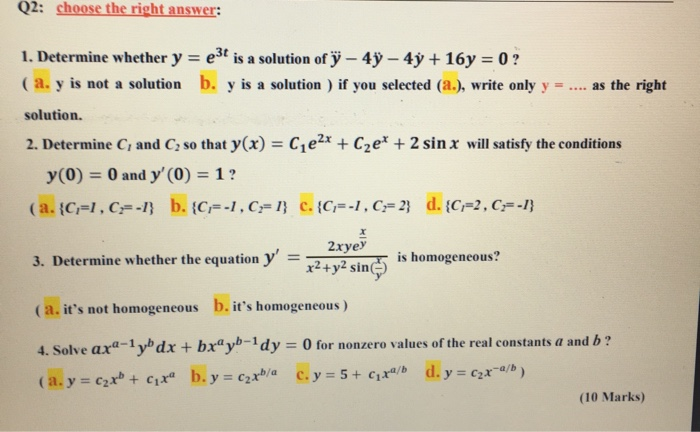Q2: choose the right answer: 1. Determine whether y = e3t is a solution of ý – 49 – 4y + 16 y = 0 ? ( a. y is not a solution b. y is a solution ) if you selected (a.), write only y = .... as the right solution. 2. Determine C, and C so that y(x) = Cje2x + Czet + 2 sin x will satisfy the conditions y(0) = 0 and y'(0) = 1? (a.{C=1,C=-1} b. {C=-1, C=1} c. {C=-1,C=2} d. {C=2, C;=-1} 3. Determine whether the equation y' 2xyey x2 + y2 sin is homogeneous? (a.it's not homogeneous b. it's homogeneous) 4. Solve axa-1 yb dx + bxºyb-1dy = 0 for nonzero values of the real constants a and b? (a. y = C2x + Cix b.y = C2xb/a C. y = 5+ Cixa/b d. y = czx-a/b) (10 Marks)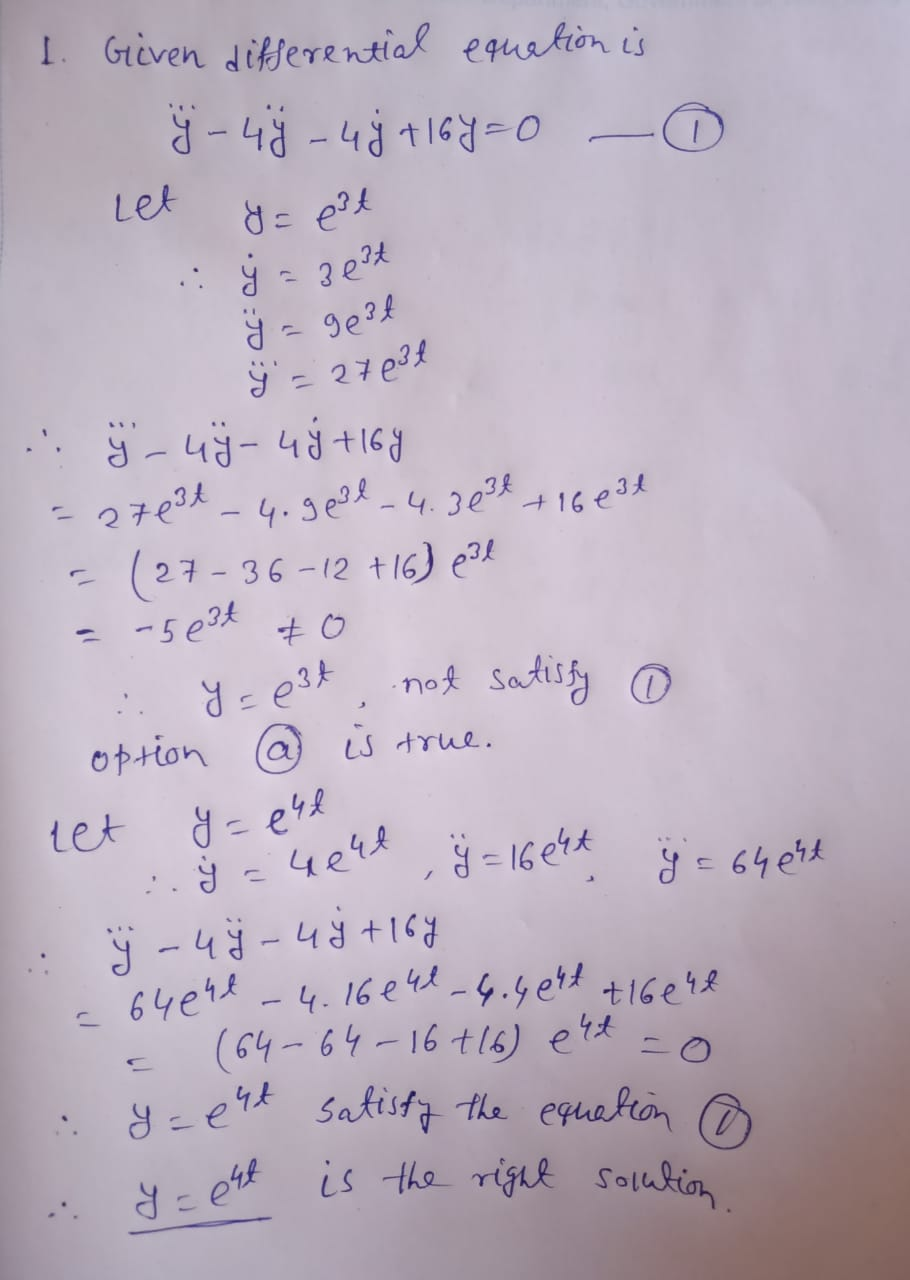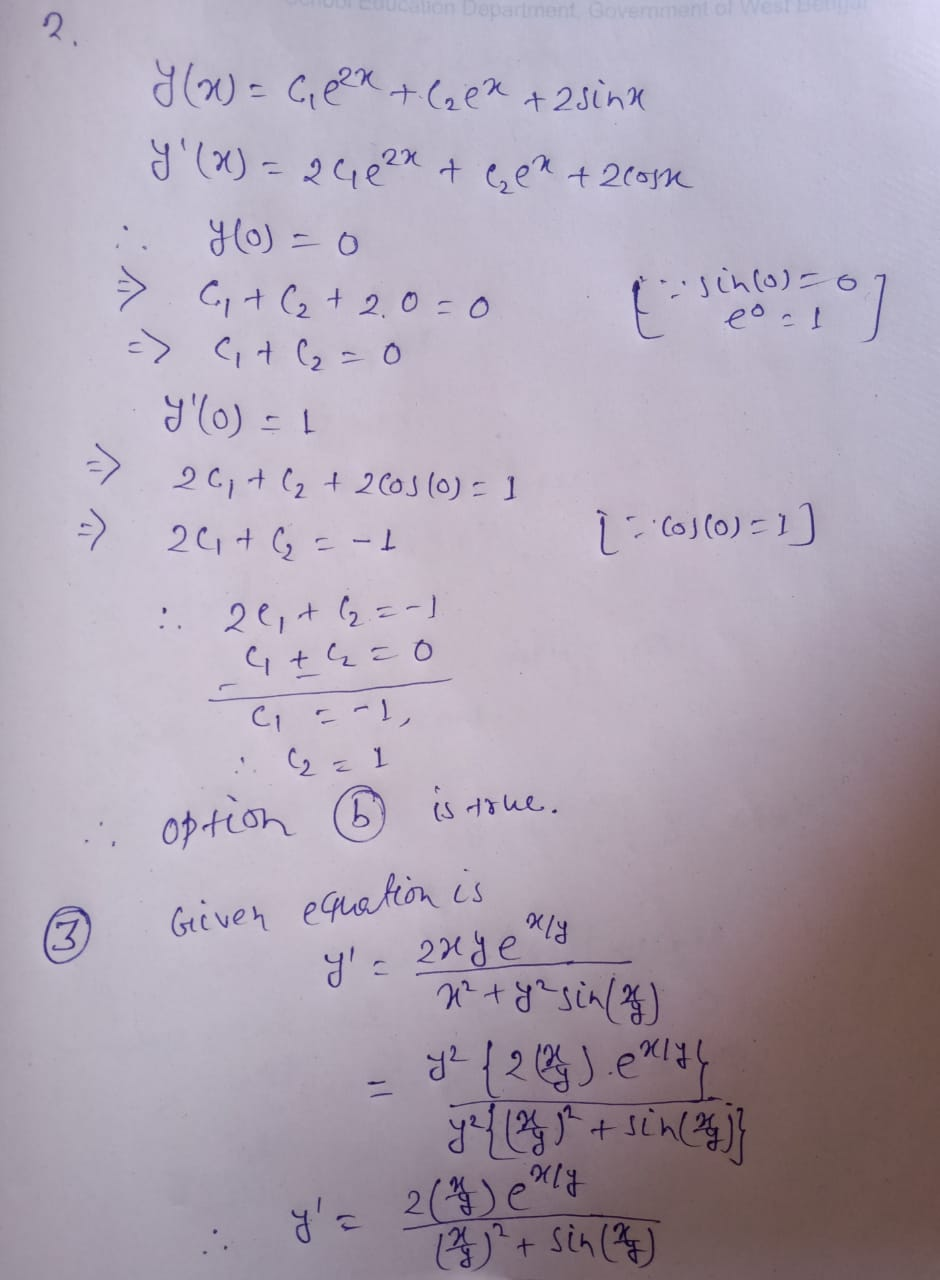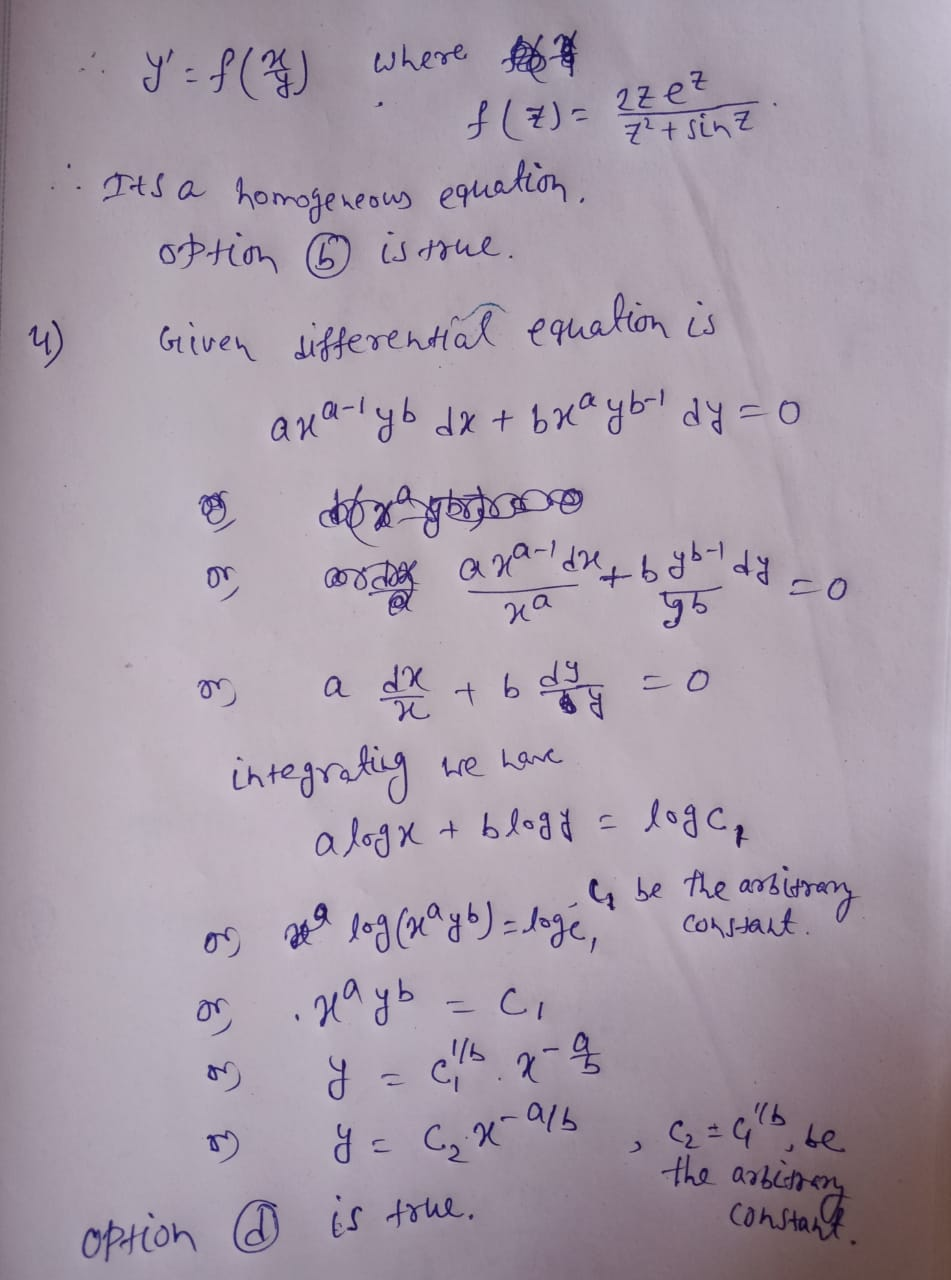##### Add Answer of: Q2: choose the right answer: 1. Determine whether y = e3t is a solution of ý...
Similar Homework Help Questions
• ### II. Determine the general solution of the given 2nd order linear homogeneous equation. 1. y" -...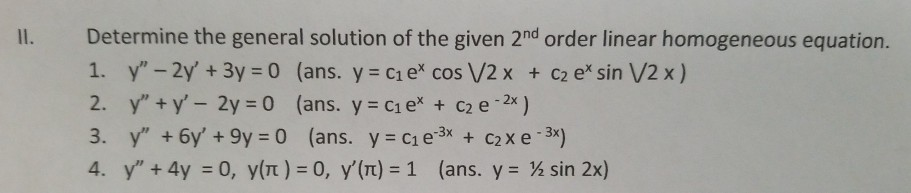II. Determine the general solution of the given 2nd order linear homogeneous equation. 1. y" - 2y' + 3y = 0 (ans. y = ci e' cos V2 x + C2 e* sin V2 x) 2. y" + y' - 2y = 0 (ans. y = C1 ex + C2 e -2x) 3. y" + 6y' + 9y = 0 (ans. y = C1 e 3x + c2x e-3x) 4. Y" + 4y = 0, y(t) = 0, y'(T) =...

• ### Question 6 The solution of the differential equation x?y" – 3xy' + 4y = 0) is...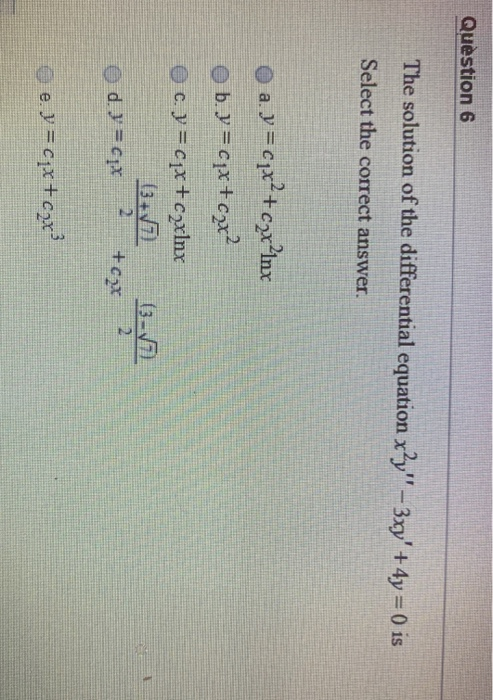Question 6 The solution of the differential equation x?y" – 3xy' + 4y = 0) is Select the correct answer. a. y = cpx?+ czx?inx b. y=cqx+cax? c. y = cqx+c2xlnx (3-5 d = 1x 6-7 +cax e. y = cix+cox?

• ### For each nonhomogeneous linear equation below, determine what the guess solution yp would be for the...

For each nonhomogeneous linear equation below, determine what the guess solution yp would be for the method of undetermined coefficients. with steps! Thank you (a) y′′ − 4y′ + 13y = 80e−3t (b) y′′−6y′+9y=(t+1)e3t (c) y′′+4y=sin(2t)+t3−1 (d) 4y′′ − 4y′ + y = 16et/2

• ### (17 points) Match the third order linear equations with their fundamental solution sets. 1. y" +...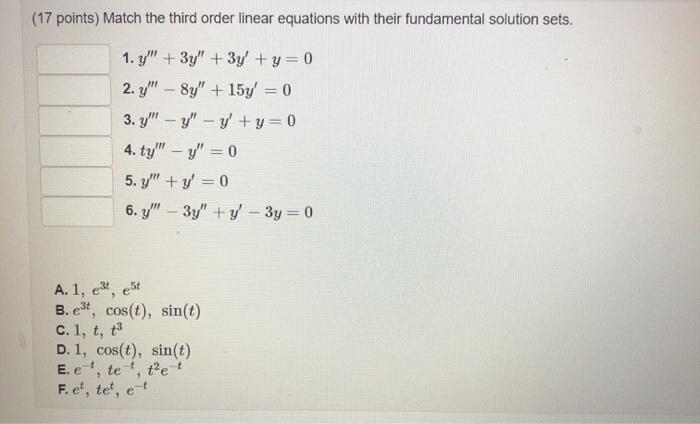(17 points) Match the third order linear equations with their fundamental solution sets. 1. y" + 3y" + 3y + y=0 2. Y" - 8y" + 15y = 0 3. y" - y" - y' + y = 0 4. ty" - y = 0 5. y" + y = 0 6. y" – 3y" + y - 3y = 0 est A. 1, e3t, B. e3t, cos(t), sin(t) c.1, t, 13 D. 1, cos(t), sin(t) E. e , te,...

• ### Q-1) Choose the right answers for the following questions: n+1 a-) + b-) + + +...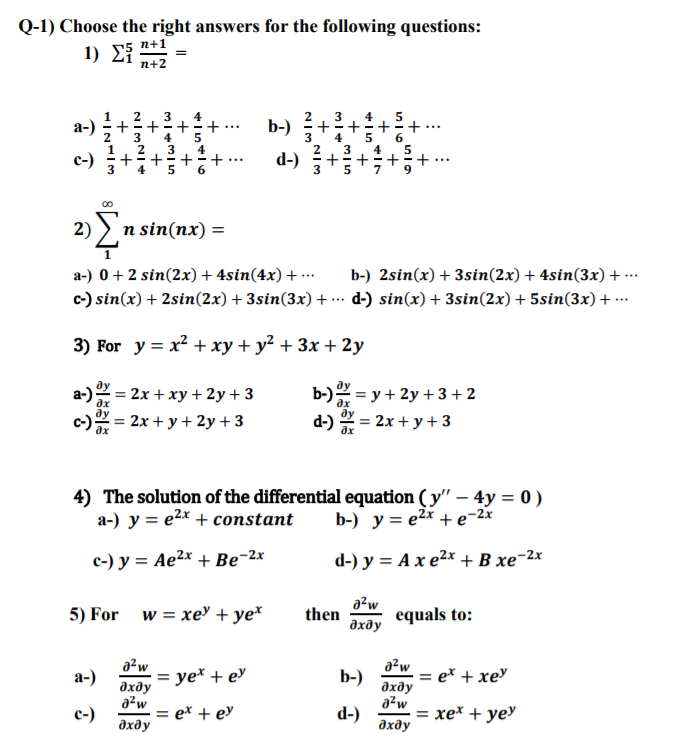Q-1) Choose the right answers for the following questions: n+1 a-) + b-) + + + + + + INWIN + + ww + + WIN WIN + ... + + ... 2) n sin(nx) = a-) 0 + 2 sin(2x) + 4sin(4x) + ... b-) 2sin(x) + 3sin(2x) + 4sin(3x) + .. C-) sin(x) + 2sin(2x) + 3sin(3x) + ... d-) sin(x) + 3sin(2x) + 5sin(3x) + ... 3) For y = x² + xy + y2 + 3x...

• ### 1. Can we obtain a general solution? 2. What techniques would you use in order? sin(t)y"...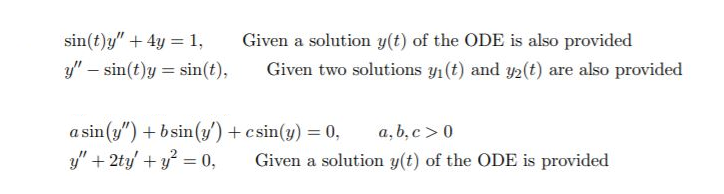1. Can we obtain a general solution? 2. What techniques would you use in order? sin(t)y" + 4y = 1, Y" - sin(t)y=sin(t), Given a solution y(t) of the ODE is also provided Given two solutions y(t) and y(t) are also provided a sin(y") + b sin(y') + csin(y) = 0, a,b,c > 0 y" + 2ty' + y2 = 0, Given a solution y(t) of the ODE is provided

• ### Question 4 4. Determine what the guess solution yp would be for the method of undetermined...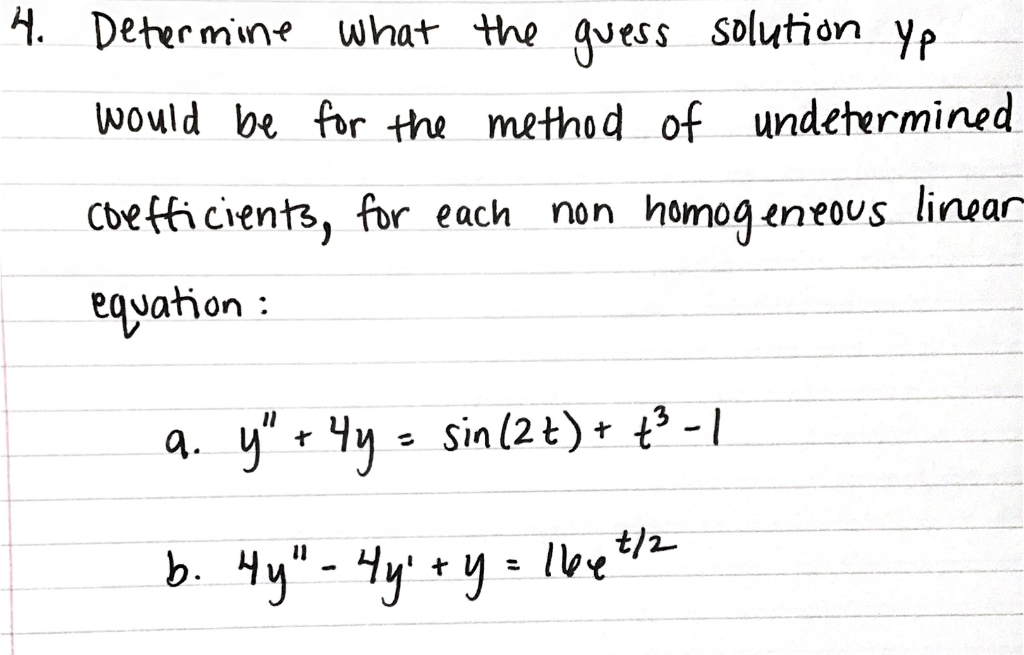Question 4 4. Determine what the guess solution yp would be for the method of undetermined Coefficients, for each non homogeneous linear equation : q. y" + 4y = sin(2t)+ + - 1 t/ b. 4y" - 4y + y = 16e

• ### Determine whether the guiven order pair is a solution of the system of equations 1

Determine whether the guiven order pair is a solution of the system of equations 1. (1,-1) 3x-2y=5 -2x+4y=6 2. (-2,3) x-y = -5 -3x+2y=12 Solve Graphically x+y=2 2x-y=1 2x+y=0 3x-5y=0 4x-2y=10 x+3y=6

• ### homo 2nd order linear equations is necessarily the number -b/2a)]. 1. Find the general solution to...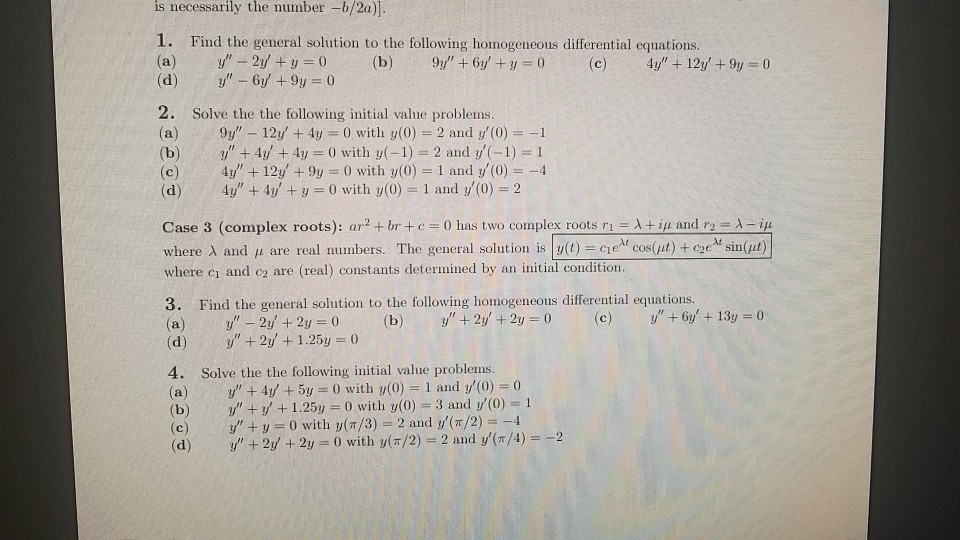homo 2nd order linear equations is necessarily the number -b/2a)]. 1. Find the general solution to the following homogeneous differential equations. (a) y" - 2y + y = 0 (b) 9y" + 6y + y = 0 (c) 4y" + 12y +9y = 0 (d) y' - 6y +9y = 0 2. Solve the the following initial value problems. (a) 9y" - 12y + 4y = 0 with y(0) = 2 and y(0) = -1 (b) y' + 4y +...

• ### (17 points) Match the third order linear equations with their fundamental solution sets. 1. y" -...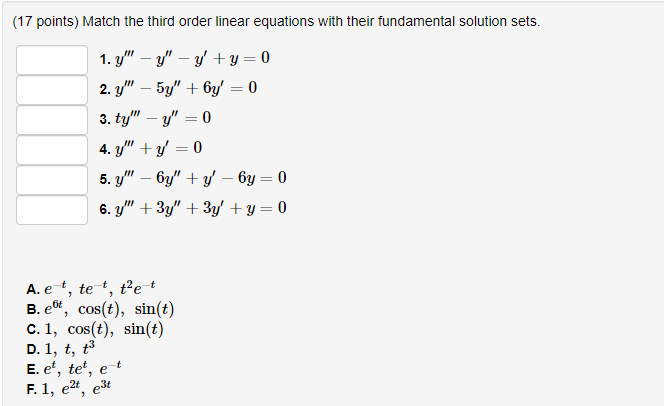(17 points) Match the third order linear equations with their fundamental solution sets. 1. y" - Y" - ' +y=0 2. y'" - 5y" + 6y' = 0 3. ty" - y" = 0 4. Y" + y 0 5.Y" - 6y" + y - by = 0 6. Y" + 3y" + 3y + y=0 A. e , te, tet B. e, cos(t), sin(t) C. 1, cos(t), sin(t) D. 1, t, 13 E. et, tet, et F. 1, 2,...

Free Homework App